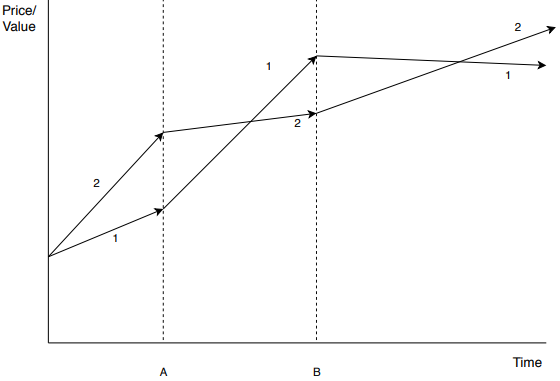## Optimized Cryptocurrency Trading Algorithms – Hacker Noon### Algorithm 2:

#### Motivation:

The weighted knapsack problem is the primary motivation behind this algorithm. I will give a small example of the weighted knapsack problem. Let us say we have to fill a knapsack that can fit a maximum of 10kgs weight with the items. Each item has a value. So we need to fill the items in such a way that we have the maximum combined value of them while not crossing the 10kg limit. I will now explain my algorithm.

#### Explanation:

In this algorithm, let us assume that we have an investor. We will suggest to him a way to invest so that he will get the maximum return on investment.

Now let us define an equation,

A*x + B*y + C*z = I

Where [A, B, C] are the number of tokens of three ICOs respectively that will be purchased by the investor and [x, y, z] are the values of these tokens. Let ‘I’ be the total money the investor is planning to invest.

Let A*X + B*Y + C*Z = O

Where [X, Y, Z] are the appreciated values of the tokens and “O” is the return on investment.

Now, we will suggest the above combination in such a way that when the price of the three tokens appreciates we get the maximum return on investment.

#### Implementation:

We can first give the investor the option to choose the number of ICOs he is planning to invest in. Once we have that number as the number, then we can give him the optimum combination for investing. In case the investor has any doubts regarding the combination, then we can get him in contact with the investment advisor of our company to help him decide. This combination can be selected with the help of the ICO data from our database. Our database must have the predicted future price of the tokens. This prediction can be achieved by the same way as I have mentioned in the implementation of my first algorithm i.e with the sentiment analysis of the ICO’s hype on its Twitter page and the data given to us by the ICO.